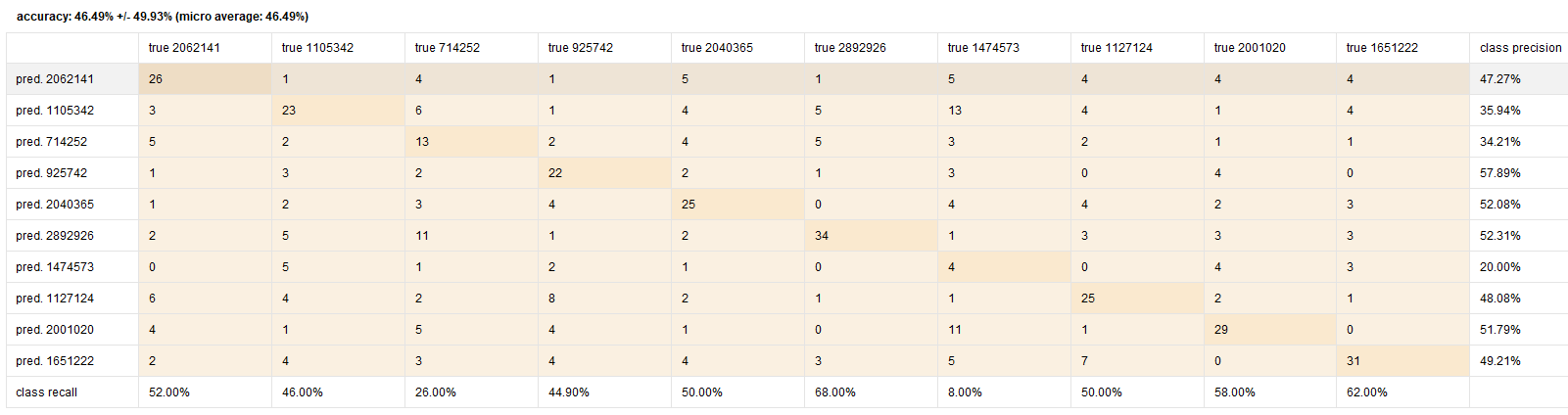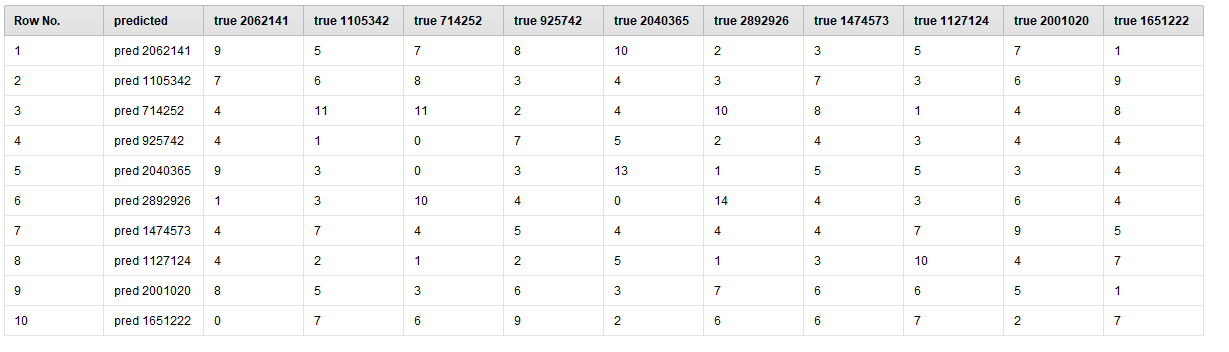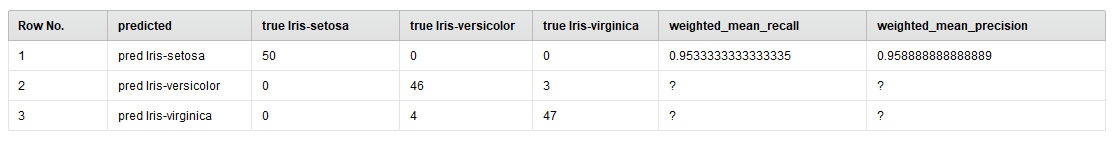# Performance operator - export results

Member Posts: 22Contributor II
edited July 2019 in Help
Hi, I have table with results:How I can save this table as excel file or csv file? Or maybe as image?

Tagged:

• Moderator, Member Posts: 999Unicorn
Hello @Serek91

There is a "performance to data" operator, you can connect this to the performance operator and this will help you connect to write excel or CSV operator. But it will save the accuracy and other parameters and not the confusion matrix.

I see that the confusion matrix can be saved using report generation extension, there are two reporting extensions, one is an old one and the new one is "Advanced reporting extension" (3rd Party). This is a paid one but not much expensive if you want to consider (Click_Here).

I tried the free one "Reporting Extension", this is free but old and I don't think it works well, I tried saving as pdf but the confusion matrices are saved in the second page. Sample XML is given below with titanic dataset. You need to have the mentioned extension to run this code.

```<?xml version="1.0" encoding="UTF-8"?><process version="9.3.001">
<context>
<input/>
<output/>
<macros/>
</context>
<operator activated="true" class="process" compatibility="9.3.001" expanded="true" name="Process">
<parameter key="logverbosity" value="init"/>
<parameter key="random_seed" value="2001"/>
<parameter key="send_mail" value="never"/>
<parameter key="process_duration_for_mail" value="30"/>
<parameter key="encoding" value="SYSTEM"/>
<process expanded="true">
<operator activated="true" class="retrieve" compatibility="9.3.001" expanded="true" height="68" name="Retrieve Titanic Training" width="90" x="45" y="85">
<parameter key="repository_entry" value="//Samples/data/Titanic Training"/>
</operator>
<operator activated="true" class="split_data" compatibility="9.3.001" expanded="true" height="103" name="Split Data" width="90" x="179" y="85">
<enumeration key="partitions">
<parameter key="ratio" value="0.7"/>
<parameter key="ratio" value="0.3"/>
</enumeration>
<parameter key="sampling_type" value="automatic"/>
<parameter key="use_local_random_seed" value="false"/>
<parameter key="local_random_seed" value="1992"/>
</operator>
<operator activated="true" class="concurrency:parallel_decision_tree" compatibility="9.3.001" expanded="true" height="103" name="Decision Tree" width="90" x="380" y="85">
<parameter key="criterion" value="gain_ratio"/>
<parameter key="maximal_depth" value="10"/>
<parameter key="apply_pruning" value="true"/>
<parameter key="confidence" value="0.1"/>
<parameter key="apply_prepruning" value="true"/>
<parameter key="minimal_gain" value="0.01"/>
<parameter key="minimal_leaf_size" value="2"/>
<parameter key="minimal_size_for_split" value="4"/>
<parameter key="number_of_prepruning_alternatives" value="3"/>
</operator>
<operator activated="true" class="apply_model" compatibility="9.3.001" expanded="true" height="82" name="Apply Model" width="90" x="514" y="187">
<list key="application_parameters"/>
<parameter key="create_view" value="false"/>
</operator>
<operator activated="true" class="performance_classification" compatibility="9.3.001" expanded="true" height="82" name="Performance (2)" width="90" x="648" y="187">
<parameter key="main_criterion" value="first"/>
<parameter key="accuracy" value="true"/>
<parameter key="classification_error" value="false"/>
<parameter key="kappa" value="false"/>
<parameter key="weighted_mean_recall" value="false"/>
<parameter key="weighted_mean_precision" value="false"/>
<parameter key="spearman_rho" value="false"/>
<parameter key="kendall_tau" value="false"/>
<parameter key="absolute_error" value="false"/>
<parameter key="relative_error" value="false"/>
<parameter key="relative_error_lenient" value="false"/>
<parameter key="relative_error_strict" value="false"/>
<parameter key="normalized_absolute_error" value="false"/>
<parameter key="root_mean_squared_error" value="false"/>
<parameter key="root_relative_squared_error" value="false"/>
<parameter key="squared_error" value="false"/>
<parameter key="correlation" value="false"/>
<parameter key="squared_correlation" value="false"/>
<parameter key="cross-entropy" value="false"/>
<parameter key="margin" value="false"/>
<parameter key="soft_margin_loss" value="false"/>
<parameter key="logistic_loss" value="false"/>
<parameter key="skip_undefined_labels" value="true"/>
<parameter key="use_example_weights" value="true"/>
<list key="class_weights"/>
</operator>
<operator activated="true" class="reporting:generate_report" compatibility="8.1.000" expanded="true" height="82" name="Generate Report" width="90" x="916" y="136">
<parameter key="report_name" value="test"/>
<parameter key="format" value="PDF"/>
<parameter key="report_to_repository" value="false"/>
<parameter key="html_output_directory" value="F:\RM"/>
<parameter key="pdf_output_file" value="F:\RM\test.pdf"/>
<parameter key="excel_output_file" value="F:\RM\report.xls"/>
<parameter key="html_template_file" value="F:\RM\test_1.html"/>
<parameter key="html_logo_file" value="F:\RM\test_1"/>
<parameter key="html_image_format" value="png"/>
<parameter key="image_col_span" value="100"/>
<parameter key="image_row_span" value="300"/>
<parameter key="page_size" value="A4"/>
<parameter key="page_format" value="portrait"/>
<parameter key="template_type" value="none"/>
<parameter key="image_alignment" value="aspect_ratio"/>
<parameter key="set_background_color" value="false"/>
<parameter key="background_color" value="255,255,255"/>
<parameter key="page_width" value="595"/>
<parameter key="page_height" value="842"/>
<parameter key="top_page_margin" value="36"/>
<parameter key="bottom_page_margin" value="36"/>
<parameter key="left_page_margin" value="36"/>
<parameter key="right_page_margin" value="36"/>
<parameter key="section_one_font_size" value="12.0"/>
<parameter key="section_one_font_style_bold" value="false"/>
<parameter key="section_one_font_style_italic" value="false"/>
<parameter key="section_one_font_style_underline" value="false"/>
<parameter key="section_one_font_style_strikethrough" value="false"/>
<parameter key="section_one_font_color" value="0,0,0"/>
<parameter key="section_two_font_size" value="12.0"/>
<parameter key="section_two_font_style_bold" value="false"/>
<parameter key="section_two_font_style_italic" value="false"/>
<parameter key="section_two_font_style_underline" value="false"/>
<parameter key="section_two_font_style_strikethrough" value="false"/>
<parameter key="section_two_font_color" value="0,0,0"/>
<parameter key="section_three_font_size" value="12.0"/>
<parameter key="section_three_font_style_bold" value="false"/>
<parameter key="section_three_font_style_italic" value="false"/>
<parameter key="section_three_font_style_underline" value="false"/>
<parameter key="section_three_font_style_strikethrough" value="false"/>
<parameter key="section_three_font_color" value="0,0,0"/>
<parameter key="section_four_font_size" value="12.0"/>
<parameter key="section_four_font_style_bold" value="false"/>
<parameter key="section_four_font_style_italic" value="false"/>
<parameter key="section_four_font_style_underline" value="false"/>
<parameter key="section_four_font_style_strikethrough" value="false"/>
<parameter key="section_four_font_color" value="0,0,0"/>
<parameter key="section_five_font_size" value="12.0"/>
<parameter key="section_five_font_style_bold" value="false"/>
<parameter key="section_five_font_style_italic" value="false"/>
<parameter key="section_five_font_style_underline" value="false"/>
<parameter key="section_five_font_style_strikethrough" value="false"/>
<parameter key="section_five_font_color" value="0,0,0"/>
<parameter key="text_content_font_size" value="12.0"/>
<parameter key="text_content_font_style_bold" value="false"/>
<parameter key="text_content_font_style_italic" value="false"/>
<parameter key="text_content_font_style_underline" value="false"/>
<parameter key="text_content_font_style_strikethrough" value="false"/>
<parameter key="text_content_font_color" value="0,0,0"/>
<parameter key="system_fonts" value="false"/>
<parameter key="directory_fonts" value="false"/>
<parameter key="table_column_number" value="8"/>
<parameter key="table_row_color_one" value="255,255,255"/>
<parameter key="table_row_color_two" value="192,192,192"/>
</operator>
<operator activated="true" class="reporting:report" compatibility="8.1.000" expanded="true" height="68" name="Report" width="90" x="1050" y="85">
<parameter key="report_name" value="test"/>
<parameter key="finalize_report" value="false"/>
<parameter key="specified" value="true"/>
<parameter key="reportable_type" value="Performance Vector"/>
<parameter key="renderer_name" value="Performance"/>
<list key="parameters"/>
<parameter key="image_width" value="800"/>
<parameter key="image_height" value="600"/>
</operator>
<connect from_op="Retrieve Titanic Training" from_port="output" to_op="Split Data" to_port="example set"/>
<connect from_op="Split Data" from_port="partition 1" to_op="Decision Tree" to_port="training set"/>
<connect from_op="Split Data" from_port="partition 2" to_op="Apply Model" to_port="unlabelled data"/>
<connect from_op="Decision Tree" from_port="model" to_op="Apply Model" to_port="model"/>
<connect from_op="Apply Model" from_port="labelled data" to_op="Performance (2)" to_port="labelled data"/>
<connect from_op="Performance (2)" from_port="performance" to_op="Generate Report" to_port="through 1"/>
<connect from_op="Generate Report" from_port="through 1" to_op="Report" to_port="reportable in"/>
<connect from_op="Report" from_port="reportable out" to_port="result 1"/>
<portSpacing port="source_input 1" spacing="0"/>
<portSpacing port="sink_result 1" spacing="0"/>
<portSpacing port="sink_result 2" spacing="0"/>
</process>
</operator>
</process>
```

Hope this helps.

• Moderator, Member Posts: 999Unicorn
Hello @mschmitz

Do we have any document that specifies extensions and their relevant operators? It would be good if there is one.

Thanks
• Administrator, Moderator, Employee, RapidMiner Certified Analyst, RapidMiner Certified Expert, University Professor Posts: 2,221RM Data Scientist
how would this look like?
BR,
Martin
- Head of Data Science Services at RapidMiner -
Dortmund, Germany
• Moderator, Member Posts: 999Unicorn
edited July 2019
Thanks for your response. I tried searching confusion in marketplace and it didn't return anything, I think the keyword is not searchable in this case.

Either we can do text (string) matching in market place, but my concern is it returns lot of operators with that string and may not be feasible. I am thinking something like an excel with extension name and their relevant operator with a one line description if possible. When ever a new extension is created, this should be updates with relevant information.

There may be other efficient ways, need to give some thought on this one.

Thanks
• Moderator, RapidMiner Certified Analyst, Member Posts: 286Unicorn
Hi @varunm1

Pretty strange and looks like some connection problem (?)... I personally never had any problem with search on the marketplace:Same results are achievable also on the marketplace web site: https://marketplace.rapidminer.com/UpdateServer/faces/index.xhtml

• Moderator, Member Posts: 999Unicorn
Ohh, that's interesting. Thanks @kypexin some how it didn't work for me when I looked for it before answering this. I will try again.

This is fine, this resolves my question @mschmitz

I will update if there are any issues.
• Member Posts: 22Contributor II
edited August 2019

"Confusion Matrix to Example Set" really helps. But still it is not enough^^

The result from this operator:So there are missing two parameters: class recall and class precision. Any ideas if something can do it? Or maybe I'm forced to fill missing parameters manually?
• Moderator, RapidMiner Certified Analyst, Member Posts: 934Unicorn
Hi @Serek91

There is an approximative solution to extract (in your case) as exampleset via the Performance to Data operator :
-  the weighted mean recall
-  the weighted mean precision

NB : These performances metrics are initialy calculated by the Performance (Classification) operator inside the CV operator (so you have to check these 2 performance metrics in the parameters of the ¨Performance operator).

Then you can "join" the exampleset with these 2 perf. metrics with the exampleset with your confusion matrix...
The resulting exampleset looks like that : (here for the Iris Dataset) :The process :

```<?xml version="1.0" encoding="UTF-8"?><process version="9.4.000-BETA">
<context>
<input/>
<output/>
<macros/>
</context>
<operator activated="true" class="process" compatibility="9.4.000-BETA" expanded="true" name="Process">
<parameter key="logverbosity" value="init"/>
<parameter key="random_seed" value="2001"/>
<parameter key="send_mail" value="never"/>
<parameter key="process_duration_for_mail" value="30"/>
<parameter key="encoding" value="SYSTEM"/>
<process expanded="true">
<operator activated="true" class="retrieve" compatibility="9.4.000-BETA" expanded="true" height="68" name="Retrieve Iris" width="90" x="45" y="85">
<parameter key="repository_entry" value="//Samples/data/Iris"/>
</operator>
<operator activated="true" class="concurrency:cross_validation" compatibility="9.4.000-BETA" expanded="true" height="145" name="Cross Validation" width="90" x="179" y="85">
<parameter key="split_on_batch_attribute" value="false"/>
<parameter key="leave_one_out" value="false"/>
<parameter key="number_of_folds" value="10"/>
<parameter key="sampling_type" value="automatic"/>
<parameter key="use_local_random_seed" value="false"/>
<parameter key="local_random_seed" value="1992"/>
<parameter key="enable_parallel_execution" value="true"/>
<process expanded="true">
<operator activated="true" class="concurrency:parallel_decision_tree" compatibility="9.4.000-BETA" expanded="true" height="103" name="Decision Tree" width="90" x="112" y="34">
<parameter key="criterion" value="gain_ratio"/>
<parameter key="maximal_depth" value="10"/>
<parameter key="apply_pruning" value="true"/>
<parameter key="confidence" value="0.1"/>
<parameter key="apply_prepruning" value="true"/>
<parameter key="minimal_gain" value="0.01"/>
<parameter key="minimal_leaf_size" value="2"/>
<parameter key="minimal_size_for_split" value="4"/>
<parameter key="number_of_prepruning_alternatives" value="3"/>
</operator>
<connect from_port="training set" to_op="Decision Tree" to_port="training set"/>
<connect from_op="Decision Tree" from_port="model" to_port="model"/>
<portSpacing port="source_training set" spacing="0"/>
<portSpacing port="sink_model" spacing="0"/>
<portSpacing port="sink_through 1" spacing="0"/>
</process>
<process expanded="true">
<operator activated="true" class="apply_model" compatibility="9.4.000-BETA" expanded="true" height="82" name="Apply Model" width="90" x="45" y="34">
<list key="application_parameters"/>
<parameter key="create_view" value="false"/>
</operator>
<operator activated="true" class="performance_classification" compatibility="9.4.000-BETA" expanded="true" height="82" name="Performance" width="90" x="179" y="34">
<parameter key="main_criterion" value="first"/>
<parameter key="accuracy" value="true"/>
<parameter key="classification_error" value="false"/>
<parameter key="kappa" value="false"/>
<parameter key="weighted_mean_recall" value="true"/>
<parameter key="weighted_mean_precision" value="true"/>
<parameter key="spearman_rho" value="false"/>
<parameter key="kendall_tau" value="false"/>
<parameter key="absolute_error" value="false"/>
<parameter key="relative_error" value="false"/>
<parameter key="relative_error_lenient" value="false"/>
<parameter key="relative_error_strict" value="false"/>
<parameter key="normalized_absolute_error" value="false"/>
<parameter key="root_mean_squared_error" value="false"/>
<parameter key="root_relative_squared_error" value="false"/>
<parameter key="squared_error" value="false"/>
<parameter key="correlation" value="false"/>
<parameter key="squared_correlation" value="false"/>
<parameter key="cross-entropy" value="false"/>
<parameter key="margin" value="false"/>
<parameter key="soft_margin_loss" value="false"/>
<parameter key="logistic_loss" value="false"/>
<parameter key="skip_undefined_labels" value="true"/>
<parameter key="use_example_weights" value="true"/>
<list key="class_weights"/>
</operator>
<connect from_port="model" to_op="Apply Model" to_port="model"/>
<connect from_port="test set" to_op="Apply Model" to_port="unlabelled data"/>
<connect from_op="Apply Model" from_port="labelled data" to_op="Performance" to_port="labelled data"/>
<connect from_op="Performance" from_port="performance" to_port="performance 1"/>
<portSpacing port="source_model" spacing="0"/>
<portSpacing port="source_test set" spacing="0"/>
<portSpacing port="source_through 1" spacing="0"/>
<portSpacing port="sink_test set results" spacing="0"/>
<portSpacing port="sink_performance 1" spacing="0"/>
<portSpacing port="sink_performance 2" spacing="0"/>
</process>
</operator>
<operator activated="true" class="multiply" compatibility="9.4.000-BETA" expanded="true" height="103" name="Multiply" width="90" x="313" y="142"/>
<operator activated="true" class="converters:confusionmatrix_2_example_set" compatibility="0.5.000" expanded="true" height="82" name="Confusion Matrix to ExampleSet" width="90" x="447" y="136"/>
<operator activated="true" class="performance_to_data" compatibility="9.4.000-BETA" expanded="true" height="82" name="Performance to Data" width="90" x="447" y="238"/>
<operator activated="true" class="select_attributes" compatibility="9.4.000-BETA" expanded="true" height="82" name="Select Attributes" width="90" x="581" y="238">
<parameter key="attribute_filter_type" value="subset"/>
<parameter key="attribute" value=""/>
<parameter key="attributes" value="Criterion|Value"/>
<parameter key="use_except_expression" value="false"/>
<parameter key="value_type" value="attribute_value"/>
<parameter key="use_value_type_exception" value="false"/>
<parameter key="except_value_type" value="time"/>
<parameter key="block_type" value="attribute_block"/>
<parameter key="use_block_type_exception" value="false"/>
<parameter key="except_block_type" value="value_matrix_row_start"/>
<parameter key="invert_selection" value="false"/>
<parameter key="include_special_attributes" value="false"/>
</operator>
<operator activated="true" class="filter_example_range" compatibility="9.4.000-BETA" expanded="true" height="82" name="Filter Example Range" width="90" x="715" y="238">
<parameter key="first_example" value="2"/>
<parameter key="last_example" value="3"/>
<parameter key="invert_filter" value="false"/>
</operator>
<operator activated="true" class="transpose" compatibility="9.4.000-BETA" expanded="true" height="82" name="Transpose" width="90" x="849" y="238"/>
<operator activated="true" class="rename_by_example_values" compatibility="9.4.000-BETA" expanded="true" height="82" name="Rename by Example Values" width="90" x="983" y="238">
<parameter key="row_number" value="1"/>
</operator>
<operator activated="true" class="select_attributes" compatibility="9.4.000-BETA" expanded="true" height="82" name="Select Attributes (2)" width="90" x="1117" y="187">
<parameter key="attribute_filter_type" value="subset"/>
<parameter key="attribute" value="id"/>
<parameter key="attributes" value="id|Criterion"/>
<parameter key="use_except_expression" value="false"/>
<parameter key="value_type" value="attribute_value"/>
<parameter key="use_value_type_exception" value="false"/>
<parameter key="except_value_type" value="time"/>
<parameter key="block_type" value="attribute_block"/>
<parameter key="use_block_type_exception" value="false"/>
<parameter key="except_block_type" value="value_matrix_row_start"/>
<parameter key="invert_selection" value="true"/>
<parameter key="include_special_attributes" value="true"/>
</operator>
<operator activated="true" class="operator_toolbox:merge" compatibility="2.1.000" expanded="true" height="103" name="Merge Attributes" width="90" x="1251" y="85">
<parameter key="handling_of_duplicate_attributes" value="rename"/>
<parameter key="handling_of_special_attributes" value="keep_first_special_other_regular"/>
<parameter key="handling_of_duplicate_annotations" value="rename"/>
</operator>
<connect from_op="Retrieve Iris" from_port="output" to_op="Cross Validation" to_port="example set"/>
<connect from_op="Cross Validation" from_port="example set" to_port="result 1"/>
<connect from_op="Cross Validation" from_port="performance 1" to_op="Multiply" to_port="input"/>
<connect from_op="Multiply" from_port="output 1" to_op="Confusion Matrix to ExampleSet" to_port="per"/>
<connect from_op="Multiply" from_port="output 2" to_op="Performance to Data" to_port="performance vector"/>
<connect from_op="Confusion Matrix to ExampleSet" from_port="exa" to_op="Merge Attributes" to_port="example set 1"/>
<connect from_op="Performance to Data" from_port="example set" to_op="Select Attributes" to_port="example set input"/>
<connect from_op="Select Attributes" from_port="example set output" to_op="Filter Example Range" to_port="example set input"/>
<connect from_op="Filter Example Range" from_port="example set output" to_op="Transpose" to_port="example set input"/>
<connect from_op="Transpose" from_port="example set output" to_op="Rename by Example Values" to_port="example set input"/>
<connect from_op="Rename by Example Values" from_port="example set output" to_op="Select Attributes (2)" to_port="example set input"/>
<connect from_op="Select Attributes (2)" from_port="example set output" to_op="Merge Attributes" to_port="example set 2"/>
<connect from_op="Merge Attributes" from_port="merged set" to_port="result 2"/>
<portSpacing port="source_input 1" spacing="0"/>
<portSpacing port="sink_result 1" spacing="0"/>
<portSpacing port="sink_result 2" spacing="0"/>
<portSpacing port="sink_result 3" spacing="0"/>
</process>
</operator>
</process>
```
Hope this helps,

Regards,

Lionel

• Member Posts: 22Contributor II
In you example Weighted Mean Recall has the same value as Accuracy. In my process, Weighted Mean Recall has exactly 10x smaller value than Accuracy. Why?
• Moderator, RapidMiner Certified Analyst, Member Posts: 934Unicorn
Hi @Serek91,

Mmmh, at first sight it is strange (but interesting) ....
I'm considering the picture you shared in your first post :
- accuracy = 46,49 %
- When I average the different class recall in your results (52 %, 46%, 26% etc.) I obtain weighted_mean_recall = 46,49 % (weights = 1, 1,1 etc.) = accuracy.
Anyways to understand what's going on, can you share please :

Regards,

Lionel

• Member Posts: 22Contributor II
edited August 2019
Process and CSV are added in attachment.

EDIT:
Even I use your solution, it is not ideal (weight mean is added to the first row, but it applies to the all data, not only one row).

I want add to the csv two more parameters: row with class recall and column with class precision. Or only with class recall (it is more important). Can I do this somehow?

EDIT2:
I think that the fastest solution will be:
1) Copy text from whole accuracy table
2) Paste to the excel file
3) Export as CSV